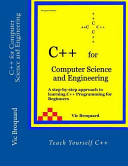August 29, 2014  |  604 pages
Undoubtedly, the best beginning book around for the novice, C++ Programming for Computer Science and Engineering is designed for CS1 and other courses covering beginning programming in C++. It is aimed at readers with little or no programming experience. C++ Programming for Computer Science and Engineering is a very readable beginning textbook. C++ Programming for Computer Science and Engineering is designed for a college level introductory C++ course for both the Computer Science and Engineering curricula. Written for the novice programmer, this book assumes no prior knowledge of computer programming. The main elements of the language are introduced step by step in a logical, gradient manner.Each chapter has three main sections. The Basics Section presents the new features of the language. This is followed by two applications sections, one geared for Computer Science majors and one for Engineering majors. Thus, the student can see solid examples of the language's application in their field. Good programming design practices are introduced early and utilized in every sample program in the book. These include Top-down Design, the Cycle of Data Processing (Input, Process, Output) and a form of elementary pseudocoding with a main storage diagram. By continuous examples, the student is shown that the optimum way to write a program is to design before you begin the actual coding into the C++ language. C++ Programming for Computer Science and Engineering contains 47 complete programs which are available ready for compilation and your experimentation. The sample programs along with a Microsoft Visual C++ .NET project for each is included with the book. The samples are of increasing sophistication and illustrate many of the basic algorithms needed by the beginning programmer. There are samples for the Basic, Computer Science and Engineering Sections. All sample programs are extensively commented so that they could be easily maintained. Generally, the Computer Science examples concentrate on the types of programs often found in this discipline as well as business data processing. They include such items as using input and output files, control break reports, summary reports, merging files of data, file update programs with emphasis on writing reusable, generic functions, sorting arrays, binary search, character string manipulation, use of structures and binary file actions. In contrast, the Engineering sample programs illustrate problems found in many different areas of engineering and numerical analysis. The basic principles of numerical analysis are presented in Chapter 5 with each chapter after that covering another analysis tool. The sample programs thus illustrate many different types of equation calculations. Covered are root solving (using the bisection method, Regula Falsi, Newton's Method and the secant method), numerical integration using the trapezoid method and Simpson's Rule, menu processing, plotting graphs, statistical computations, Least Squares Curve Fitting, matrix math operations, Gauss and Gauss-Jordan methods for solving equations and the use of structures to simplify parameter passing. Note that many of the engineering samples can also be profitably covered in a Computer Science course and vice versa.
Synopsis:
Title:
Author:
Release Date:
Page Count:
Series Name:
#
Cover Image:
(Must be JPEG and less than 1 MB)